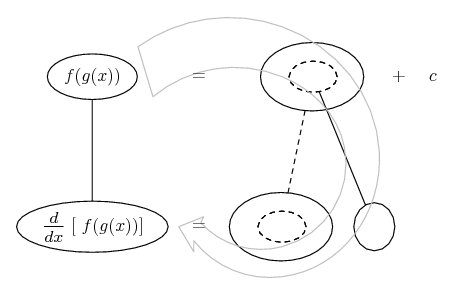# Thread: how to integrate this?

1. ## how to integrate this?

How to integrate this problem?

I don't know where should I start with this one.

$\displaystyle \int\frac{dx}{\tan2x[1-2lnsin2x]}$

2. Hint: $\displaystyle \displaystyle \left(1-2\ln{\sin{2x}}\right)' = \frac{-4}{\tan{2x}}$.

3. substitution $\displaystyle t=1-2\ln(\sin 2x)$ will solve the problem.

4. Can you make it more easier for me to understand?I have to use logarithmic formula in that given right?

5. They have practically solved the problem for you. No, they cannot make it clearer.

If $\displaystyle t=1-2\ln(\sin 2x)$ (the substitution Krizalid suggests), what is dt? (TheCoffeeMachine has already answered that.)

6. Just in case a picture helps...

As people have hinted...... i.e., notice how to differentiate $\displaystyle 1 - 2\ln(\sin2x)$ wrt x. I.e. consider how to differentiate $\displaystyle 1 - 2\ln t$ wrt t, or the corresponding balloon here, wrt the dashed balloon...But then apply the chain rule, where (key in spoiler) ...

Spoiler:... is the chain rule. Straight continuous lines differentiate downwards (integrate up) with respect to the main variable (in this case ), and the straight dashed line similarly but with respect to the dashed balloon expression (the inner function of the composite which is subject to the chain rule).

The general drift is...So we get...And notice that for this we also used...Now for the integration... we want to travel up instead of down. Can you anti-differentiate

$\displaystyle \frac{-1}{4t}$

wrt t? I.e...If so then you're ready to fill out the rest of this..._________________________________________

Don't integrate - balloontegrate!

Balloon Calculus; standard integrals, derivatives and methods

Balloon Calculus Drawing with LaTeX and Asymptote!

#### Search Tags

integrate